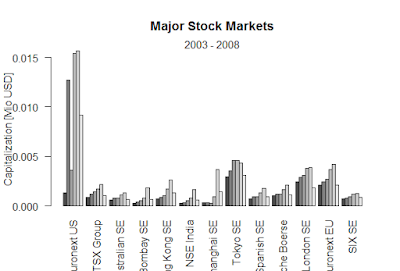Showing posts with label log to one vector. Show all posts
Showing posts with label log to one vector. Show all posts

## VECTORS structure in R is the vector.

### A vector is an object that consists of a number of elements of the same type, for example all doubles or all logical, as is called an atomic object.

> x <- c(10,2,15,35)
> x
 10  2 15 35
> y<- c(x,0.55.x,x)
The function c merges an arbitrary number of vectors to one vector. A single number is regarded as a vector of length one.
> y<- c(x,0.55,x,x)
> y
 10.00  2.00 15.00 35.00  0.55 10.00  2.00 15.00 35.00 10.00  2.00
 15.00 35.00
> z <-c(x+1,9,x+0.5,7,x+5)
> z
 11.0  3.0 16.0 36.0  9.0 10.5  2.5 15.5 35.5  7.0 15.0  7.0 20.0
 40.0
Use the function round to round the numbers in a vector
> round (z,4)
 11.0  3.0 16.0 36.0  9.0 10.5  2.5 15.5 35.5  7.0 15.0  7.0 20.0
 40.0
> p <-x*x
> p
  100    4  225 1225
> log(x)
 2.3025851 0.6931472 2.7080502 3.5553481

### Black-Scholes formula-R

Black-Scholes formula-R > BlackScholes <- function(TypeFlag = c("c", "p"), S, X, Time, r, b, sigma) { TypeFla...